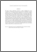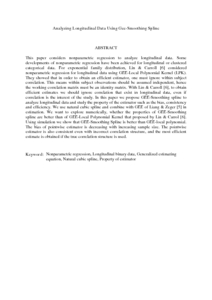# Analyzing Longitudinal Data Using Gee-Smoothing Spline

## Citation

Ibrahim, Noor Akma and Suliadi, (2010) Analyzing Longitudinal Data Using Gee-Smoothing Spline. WSEAS Transactions on Systems and Control . ISSN 1790-5117

## Abstract

This paper considers nonparametric regression to analyze longitudinal data. Some developments of nonparametric regression have been achieved for longitudinal or clustered categorical data. For exponential family distribution, Lin & Carroll  considered nonparametric regression for longitudinal data using GEE-Local Polynomial Kernel (LPK). They showed that in order to obtain an efficient estimator, one must ignore within subject correlation. This means within subject observations should be assumed independent, hence the working correlation matrix must be an identity matrix. With Lin & Carroll , to obtain efficient estimates we should ignore correlation that exist in longitudinal data, even if correlation is the interest of the study. In this paper we propose GEE-Smoothing spline to analyze longitudinal data and study the property of the estimator such as the bias, consistency and efficiency. We use natural cubic spline and combine with GEE of Liang & Zeger  in estimation. We want to explore numerically, whether the properties of GEE-Smoothing spline are better than of GEE-Local Polynomial Kernel that proposed by Lin & Carrol . Using simulation we show that GEE-Smoothing Spline is better than GEE-local polynomial. The bias of pointwise estimator is decreasing with increasing sample size. The pointwise estimator is also consistent even with incorrect correlation structure, and the most efficient estimate is obtained if the true correlation structure is used.Preview
PDF (Abstract)
Analyzing Longitudinal Data Using Gee.pdfView Item